Next: Stability of Single-Phase Substance Up: Multi-Phase Systems Previous: Equilibrium of Constant-Temperature System

# Equilibrium of Constant-Temperature Constant-Pressure System

Another case of physical interest is that where a system,, is maintained under conditions of both constant temperature and constant pressure. This is a situation that occurs frequently in the laboratory, where we might carry out an experiment in a thermostat at atmospheric pressure (say). Therefore, let us suppose thatis in thermal contact with a heat reservoir,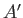, that is held at the constant temperature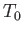, and at the constant pressure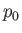. The systemcan exchange heat with the reservoir,, but the latter is so large that its temperature,, remains constant. Likewise, the systemcan change its volume,, at the expense of that of the reservoir,, doing work on the reservoir in the process. However,is so large that its pressure,, remains unaffected by this relatively small volume change.

The analysis of the equilibrium conditions for systemfollows similar lines to the that in the previous section. The entropy,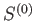, of the combined system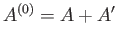satisfies the condition that in any spontaneous process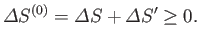(9.19)

Ifabsorbs heat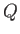fromin this process then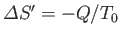. Furthermore, the first law of thermodynamics yields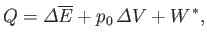(9.20)

where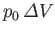is the work done byagainst the constant pressure,, of the reservoir,, and where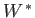denotes any other work done byin the process. (For example,might refer to electrical work.) Thus, we can write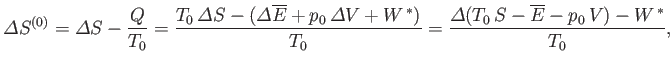(9.21)

or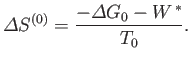(9.22)

Here, we have made use of the fact thatandare constants. We have also introduced the definition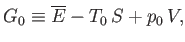(9.23)

where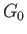becomes the Gibbs free energy,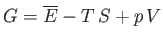, of systemwhen the temperature and pressure of this system are both equal to those of the reservoir,.

The fundamental condition (9.19) can be combined with Equation (9.22) to give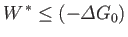(9.24)

(assuming thatis positive). This relation implies that the maximum work (other than the work done on the pressure reservoir) that can be done by systemis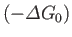. (Incidentally, this is the reason that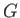is also called a free energy''.) The maximum work corresponds to the equality sign in the preceding equation, and is obtained when the process used is quasi-static (so thatis always in equilibrium with, and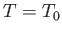,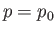).

If the external parameters of system(except its volume) are held constant then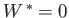, and Equation (9.24) yields the condition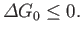(9.25)

Thus, we arrive at the following statement:
If a system is in contact with a reservoir at constant temperature and pressure then the stable equilibrium state is such that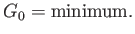The preceding statement can again be phrased in more explicit statistical terms. Suppose that the external parameters of(except its volume) are fixed, so that. Furthermore, letbe described by some parameter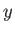. Ifchanges from some standard value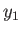then the corresponding change in the total entropy of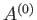is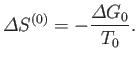(9.26)

But, in an equilibrium state, the probability,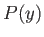, that the parameter lies betweenand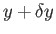is given by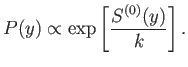(9.27)

Now, from Equation (9.26),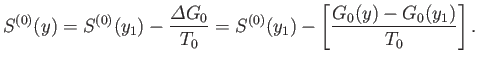(9.28)

However, becauseis just some arbitrary constant, the corresponding constant terms can be absorbed into the constant of proportionality in Equation (9.27), which then becomes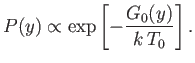(9.29)

This equation shows explicitly that the most probable state is one in whichattains a minimum value, and also allows us to determine the relative probability of fluctuations about this state.Next: Stability of Single-Phase Substance Up: Multi-Phase Systems Previous: Equilibrium of Constant-Temperature System
Richard Fitzpatrick 2016-01-25